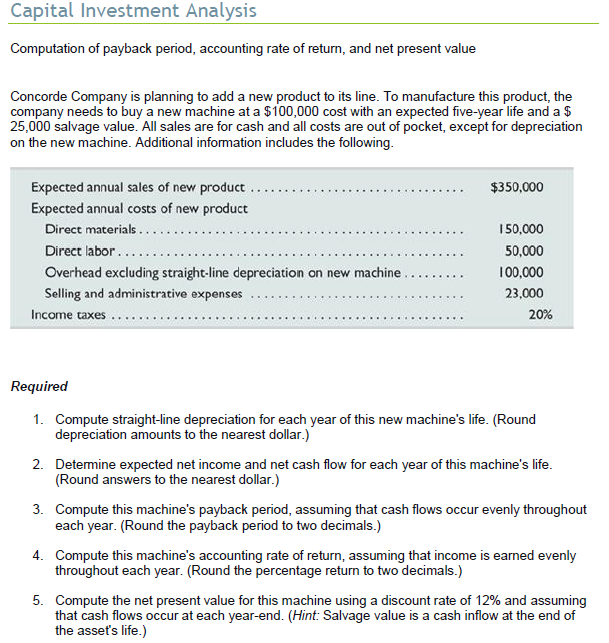# Capital Investment Analysis

\$1.00Text: Computation of payback period, accounting rate of return, and net present value Concorde Company is planning to add a new product to its line. To manufacture this product, the company needs to buy a new machine at a \$100,000 cost with an expected five-year life and a \$ 25,000 salvage value. All sales are for cash and all costs are out of pocket, except for depreciation on the new machine. Additional information includes the following. Required 1. Compute straight-line depreciation for each year of this new machine’s life. (Round depreciation amounts to the nearest dollar.) 2. Determine expected net income and net cash flow for each year of this machine’s life. (Round answers to the nearest dollar.) 3. Compute this machines payback period, assuming that cash flows occur evenly throughout each year. (Round the payback period to two decimals.) 4. Compute this machine’s accounting rate of return, assuming that income is earned evenly throughout each year. (Round the percentage return to two decimals.) 5. Compute the net present value for this machine using a discount rate of 12% and assuming that cash flows occur at each year-end. (Hint: Salvage value is a cash inflow at the end of the asset’s life.)

SKU: capital-investment-analysis Category: